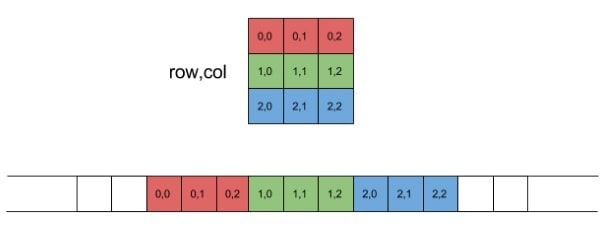# Memory Layout of a 2D Array

An image is essentially a 2 dimensional (2D) array where every element of that array represents a pixel. The value of that element determines the corresponding pixel appearance such as color or brightness (for grayscale images).

In order to process a 2D array in assembly, it’s important to understand how a 2D array is laid out in memory. First, some notes on the nomenclature of this handout. Computer memory will be represented as a linear array with low addresses on the left and high addresses on the right. Also, we're going to use programmer notation for matrices: rows and columns start with zero, at the top-left corner of the matrix. Row indices go over rows from top to bottom; column indices go over columns from left to right.

The elements sit in the memory in a manner called row-major layout where the first row of the matrix is placed in contiguous memory, then the second, and so on:Another way to describe row-major layout is that column indices change the fastest. This should be obvious by looking at the linear layout at the bottom of the diagram. If you read the element index pairs from left to right, you'll notice that the column index changes all the time, and the row index only changes once per row.

For programmers, another important observation is that given a row index (row_idx) and a column index (col_idx), the offset of the element they denote in the linear representation is:

offset = row_idx * num_of_columns + col_idx

Where num_of_columns is the number of columns per row in the matrix. It's easy to see this equation fits the linear layout in the diagram shown above.

Having the memory address of the base of an array and a pair of (row, col) indices, we are able to find the address of individual elements in a 2D array: